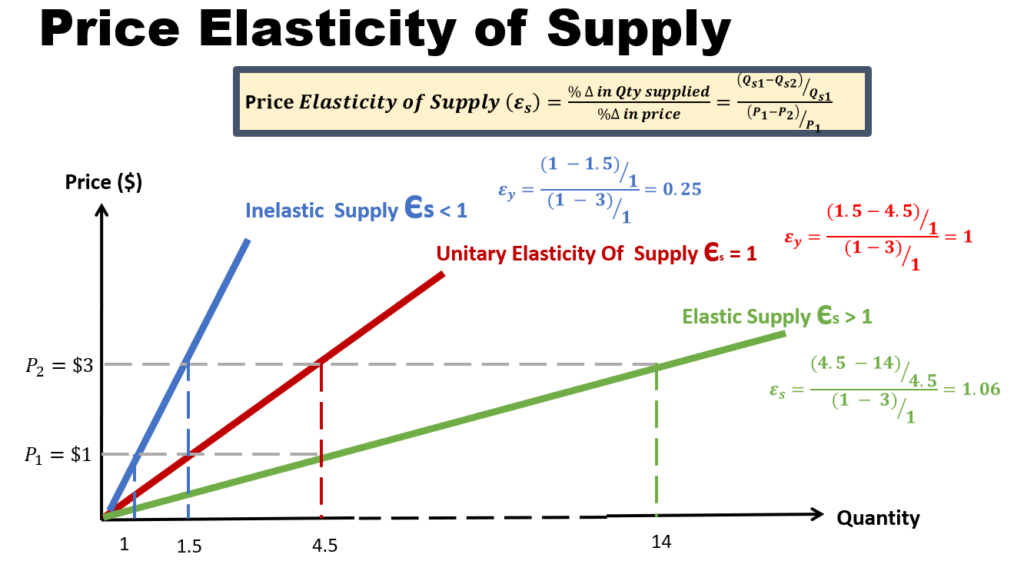# Price Elasticity of Supply

## Price Elasticity of Supply - how much quantity supplied changes over changes in price

Price elasticity of supply (Es) can be defined as the degree of responsiveness of quantity supplied to changes in price, ceteris paribus. The formula for Es is as shown in the diagram.

• If Es is between 0 and 1, then it is considered as inelastic.
• If Es is greater than 1, then it is considered as elastic.## Factors that affect elasticity of supply

### 1. Spare Capacity

• Spare capacity refers to under-utilized resources
• Higher the spare capacity of firm, greater the elasticity

### 2. Stocks

• Higher the stocks, greater the elasticity.
• This is because the firm is then able to response to a change in demand quickly by supplying these stocks to the market.

### 3. Ease of Factor Substitution

• If factors or production (Labour, capital) are easily available, then the elasticity of supply will be high.
• On example of factor is labour. In this case, ease of factor substitution means that it is easy for labour to switch from other industries into this industry.

### 4. Time Period

• The longer the time period, it means that a firm can then more easily adjust its production.
• For instance, over time, a firm may have more resources at its disposal (e.g. factors, new technology, new alternatives). The firm can then more easily adjust the quantity of goods supplied in response to the price changes.  Hence elasticity of supply will be high.